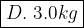## Question 5 of 10 A 15 N force is applied to an object, which then accelerates at 5.0 m/s2. What is the mass of the object?

Question

Question 5 of 10
A 15 N force is applied to an object, which then accelerates at 5.0 m/s2. What
is the mass of the object?
A. 10 kg
B. 20 kg
C. 75 kg
D. 3.0 kg

in progress 0
7 months 2021-07-13T19:42:58+00:00 2 Answers 3 views 0

1. D is the correct answer

2. Hi there!Use the following equation to solve:

F = ma, where:

F = Force (N)

m = mass (kg)

a = acceleration (m/s²)

Thus:

15 = 5m

15/5 = m

mass = 3 kg